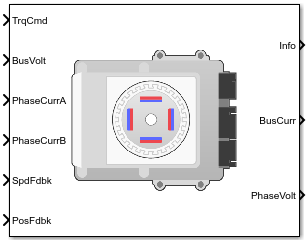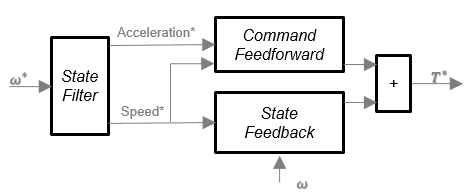# Flux-Based PM Controller

Controller for a flux-based permanent magnet synchronous motor

• Library:
• Powertrain Blockset / Propulsion / Electric Motor Controllers

•## Description

The Flux Based PM Controller block implements a flux-based, field-oriented controller for an interior permanent magnet synchronous motor (PMSM) with an optional outer-loop speed controller. The internal torque control implements strategies for achieving maximum torque per ampere (MTPA) and weakening the magnetic flux. You can specify either the speed or torque control type.

The Flux Based PM Controller implements equations for speed control, torque determination, regulators, transforms, and motors.

The figure illustrates the information flow in the block.The block implements equations using these variables.

 ω Rotor speed ω* Rotor speed command T* Torque command id i*d d-axis current d-axis current command iq i*q q-axis current q-axis current command vd, v*d d-axis voltage d-axis voltage command vq v*q q-axis voltage q-axis voltage command va, vb, vc Stator phase a, b, c voltages ia, ib, ic Stator phase a, b, c currents

### Speed Controller

To implement the speed controller, select the Control Type parameter `Speed Control`. If you select the Control Type parameter ```Torque Control```, the block does not implement the speed controller.

The speed controller determines the torque command by implementing a state filter, and calculating the feedforward and feedback commands. If you do not implement the speed controller, input a torque command to the Flux Based PM Controller block.State Filter

The state filter is a low-pass filter that generates the acceleration command based on the speed command. The discrete form of characteristic equation is given by:

`$z+{K}_{sf}{T}_{sm}-1$`

The filter calculates the gain using this equation.

`${K}_{sf}=\frac{1-\mathrm{exp}\left(-{T}_{sm}2\pi E{V}_{sf}\right)}{{T}_{sm}}$`

The equations use these variables.

 EVsf Bandwidth of the speed command filter Tsm Motion controller sample time Ksf Speed regulator time constant

State Feedback

To generate the state feedback torque, the block uses the filtered speed error signal from the state filter. To filter the speed, the block uses a proportional integral (PI) controller.

`${T}_{cmd}=K{p}_{\omega }\left({\omega }_{m}^{*}-{\omega }_{m}\right)+K{i}_{\omega }\frac{z{T}_{sm}}{z-1}\left({\omega }_{m}^{*}-{\omega }_{m}\right)$`

The equations use these variables.

 ωm Rotor speed ω*m Rotor speed command Tcmd Torque command Kpω Speed regulator proportional gain Kiω Speed regulator integral gain Tsm Speed regulator sample rate

Command Feedforward

To generate the state feedforward torque, the block uses the filtered speed and acceleration from the state filter. Also, the feedforward torque calculation uses the inertia, viscous damping, and static friction. To achieve zero tracking error, the torque command is the sum of the feedforward and feedback torque commands.

The feedforward torque command uses this equation.

where:

 Jp Rotor inertia Tcmd_ff Torque command feedforward Fs Static friction torque constant Fv Viscous friction torque constant Fs Static friction torque constant ωm Rotor speed

Current Command

The block uses lookup tables to determine the d-axis and q-axis current commands. The lookup tables are functions of mechanical speed and torque. To determine the lookup tables, you can use an external finite element analysis (FEA) models or dynamometer test results.

`$\begin{array}{l}{i}_{dref}=f\left(|{\omega }_{m}|,|{T}_{ref}|\right)\\ {i}_{qref}=sign\left({T}_{ref}\right)\ast f\left(|{\omega }_{m}|,|{T}_{ref}|\right)\end{array}$`

The equations use these variables.

 ωm Rotor speed Tref Torque command idref, iqref d- and q-axis reference current, respectively

Voltage Command

The block uses these equations to calculate the voltage in the motor reference frame.

`$\begin{array}{l}{v}_{d}=\frac{d{\psi }_{d}}{dt}+{R}_{s}{i}_{d}-{\omega }_{e}{\psi }_{q}\\ {v}_{q}=\frac{d{\psi }_{q}}{dt}+{R}_{s}{i}_{q}+{\omega }_{e}{\psi }_{d}\end{array}$`

`$\begin{array}{l}\frac{d{\psi }_{d}}{dt}+{R}_{s}{i}_{d}=K{p}_{d}\left({i}_{d}^{*}-{i}_{d}\right)+K{i}_{d}\frac{z{T}_{st}}{z-1}\left({i}_{d}^{*}-{i}_{d}\right)\\ \frac{d{\psi }_{q}}{dt}+{R}_{s}{i}_{q}=K{p}_{q}\left({i}_{q}^{*}-{i}_{q}\right)+K{i}_{q}\frac{z{T}_{st}}{z-1}\left({i}_{q}^{*}-{i}_{q}\right)\\ \\ {v}_{d}=K{p}_{i}\left({i}_{d}^{*}-{i}_{d}\right)+K{i}_{d}\frac{z{T}_{st}}{z-1}\left({i}_{d}^{*}-{i}_{d}\right)+{\omega }_{e}{\psi }_{q}\\ {v}_{q}=K{p}_{i}\left({i}_{q}^{*}-{i}_{q}\right)+K{i}_{q}\frac{z{T}_{st}}{z-1}\left({i}_{q}^{*}-{i}_{q}\right)-{\omega }_{e}{\psi }_{d}\\ \\ {\psi }_{q}=f\left({i}_{d},{i}_{q}\right)\\ {\psi }_{d}=f\left({i}_{d},{i}_{q}\right)\end{array}$`

The equations use these variables.

 ωm Rotor mechanical speed ωe Rotor electrical speed Rs, Rr Resistance of the stator and rotor windings, respectively iq, id q- and d-axis current, respectively vq, vd q- and d-axis voltage, respectively Ψq, Ψd q- and d-axis magnet flux, respectively Tst Current regulator sample rate Kid , Kiq d- and q- axis integral gain, respectively Kpd , Kpq d- and q- axis proportional gain, respectively

### Transforms

To calculate the voltages and currents in balanced three-phase (a, b) quantities, quadrature two-phase (α, β) quantities, and rotating (d, q) reference frames, the block uses the Clarke and Park Transforms.

In the transform equations.

TransformDescriptionEquations

Clarke

Converts balanced three-phase quantities (a, b) into balanced two-phase quadrature quantities (α, β).

Park

Converts balanced two-phase orthogonal stationary quantities (α, β) into an orthogonal rotating reference frame (d, q).

Inverse Clarke

Converts balanced two-phase quadrature quantities (α, β) into balanced three-phase quantities (a, b).

Inverse Park

Converts an orthogonal rotating reference frame (d, q) into balanced two-phase orthogonal stationary quantities (α, β).

The transforms use these variables.

 ωm Rotor speed P Rotor pole pairs ωe Rotor electrical speed Θe Rotor electrical angle x Phase current or voltage

### Motor

The block uses the phase currents and phase voltages to estimate the DC bus current. Positive current indicates battery discharge. Negative current indicates battery charge.

The block uses these equations.

 Load power Source power DC bus current Estimated rotor torque ${T}_{e}=1.5P\left[{\psi }_{d}{i}_{q}-{\psi }_{q}{i}_{d}\right]$ Power loss for single efficiency source to load $Pw{r}_{Loss}=\frac{100-Eff}{Eff}\cdot L{d}_{Pwr}$ Power loss for single efficiency load to source $Pw{r}_{Loss}=\frac{100-Eff}{100}\cdot |L{d}_{Pwr}|$ Power loss for tabulated efficiency

The equations use these variables.

 va, vb, vc Stator phase a, b, c voltages vbus Estimated DC bus voltage ia, ib, ic Stator phase a, b, c currents ibus Estimated DC bus current Eff Overall inverter efficiency ωm Rotor mechanical speed Lq, Ld q- and d-axis winding inductance, respectively Ψq, Ψd q- and d-axis magnet flux, respectively iq, id q- and d-axis current, respectively λ Permanent magnet flux linkage P Rotor pole pairs

### Electrical Losses

To specify the electrical losses, on the Electrical Losses tab, for Parameterize losses by, select one of these options.

SettingBlock Implementation
`Single efficiency measurement`

Electrical loss calculated using a constant value for inverter efficiency.

`Tabulated loss data`

Electrical loss calculated as a function of motor speeds and load torques.

`Tabulated efficiency data`

Electrical loss calculated using inverter efficiency that is a function of motor speeds and load torques.

• Converts the efficiency values you provide into losses and uses the tabulated losses for simulation.

• Ignores efficiency values you provide for zero speed or zero torque. Losses are assumed zero when either torque or speed is zero.

• Uses linear interpolation to determine losses. Provide tabulated data for low speeds and low torques, as required, to get the desired level of accuracy for lower power conditions.

• Does not extrapolate loss values for speed and torque magnitudes that exceed the range of the table.

For best practice, use `Tabulated loss data` instead of `Tabulated efficiency data`:

• Efficiency becomes ill defined for zero speed or zero torque.

• You can account for fixed losses that are still present for zero speed or torque.

## Ports

### Input

expand all

Rotor speed command, ω*m, in rad/s.

#### Dependencies

To create this port, select ```Speed Control``` for the Control Type parameter.

Torque command, T*, in N·m.

#### Dependencies

To create this port, select ```Torque Control``` for the Control Type parameter.

DC bus voltage, vbus, in V.

Stator current phase a, ia, in A.

Stator current phase b, ib, in A.

Rotor electrical angle, Θm, in rad.

### Output

expand all

Bus signal containing these block calculations.

SignalDescriptionUnits

`SrcPwr`

Source power

W

`LdPwr`

W

`PwrLoss`

Power loss

W

`MtrTrqEst`

Estimated motor torque

N·m

Estimated DC bus current, ibus, in A.

Stator terminal voltages, Va, Vb, and Vc, in V.

## Parameters

expand all

Block Options

If you select `Torque Control`, the block does not implement the speed controller.

This table summarizes the port configurations.

Port ConfigurationCreates Ports
`Speed Control`

`SpdReq`

`Torque Control`

`TrqCmd`

Motor Parameters

Motor pole pairs, P.

d-axis current, id_index, in A.

q-axis current, iq_index, in A.

d-axis flux, λd, in Wb.

q-axis flux, λq, in Wb.

Current Controller

Torque control sample time, Tst, in s.

d-axis proportional gain, Kpd, in V/A.

q-axis proportional gain, Kpq, in V/A.

d-axis integral gain, Kid, in V/A·s.

q- axis integral gain, Kiq, in V/A·s.

Torque breakpoints, Tbp, in N·m.

d-axis reference current, idref, in A.

q-axis reference current, iqref, in A.

Speed Controller

Speed regulator time constant, Ksf, in 1/s.

#### Dependencies

To enable this parameter, for the Control Type parameter, select `Speed Control`.

#### Dependencies

To enable this parameter, for the Control Type parameter, select `Speed Control`.

#### Dependencies

To enable this parameter, for the Control Type parameter, select `Speed Control`.

Inertia compensation, in kg·m^2.

#### Dependencies

To enable this parameter, for the Control Type parameter, select `Speed Control`.

Static friction, in N·m.

#### Dependencies

To enable this parameter, for the Control Type parameter, select `Speed Control`.

#### Dependencies

To enable this parameter, for the Control Type parameter, select `Speed Control`.

Electrical Losses

SettingBlock Implementation
`Single efficiency measurement`

Electrical loss calculated using a constant value for inverter efficiency.

`Tabulated loss data`

Electrical loss calculated as a function of motor speeds and load torques.

`Tabulated efficiency data`

Electrical loss calculated using inverter efficiency that is a function of motor speeds and load torques.

• Converts the efficiency values you provide into losses and uses the tabulated losses for simulation.

• Ignores efficiency values you provide for zero speed or zero torque. Losses are assumed zero when either torque or speed is zero.

• Uses linear interpolation to determine losses. Provide tabulated data for low speeds and low torques, as required, to get the desired level of accuracy for lower power conditions.

• Does not extrapolate loss values for speed and torque magnitudes that exceed the range of the table.

For best practice, use `Tabulated loss data` instead of `Tabulated efficiency data`:

• Efficiency becomes ill defined for zero speed or zero torque.

• You can account for fixed losses that are still present for zero speed or torque.

Overall inverter efficiency, Eff, in %.

#### Dependencies

To enable this parameter, for Parameterize losses by, select `Tabulated loss data`.

Speed breakpoints for lookup table when calculating losses, in rad/s.

#### Dependencies

To enable this parameter, for Parameterize losses by, select `Tabulated loss data`.

Torque breakpoints for lookup table when calculating losses, in N·m.

#### Dependencies

To enable this parameter, for Parameterize losses by, select `Tabulated loss data`.

Array of values for electrical losses as a function of `M` speeds and `N` torques, in W. Each value specifies the losses for a specific combination of speed and torque. The matrix size must match the dimensions defined by the speed and torque vectors.

#### Dependencies

To enable this parameter, for Parameterize losses by, select `Tabulated loss data`.

Speed breakpoints for lookup table when calculating efficiency, in rad/s.

#### Dependencies

To enable this parameter, for Parameterize losses by, select `Tabulated efficiency data`.

Torque breakpoints for lookup table when calculating efficiency, in N·m.

#### Dependencies

To enable this parameter, for Parameterize losses by, select `Tabulated efficiency data`.

Array of efficiency as a function of `M` speeds and `N` torque, in %. Each value specifies the efficiency for a specific combination of speed and torque. The matrix size must match the dimensions defined by the speed and torque vectors.

The block ignores efficiency values for zero speed or zero torque. Losses are zero when either torque or speed is zero. The block uses linear interpolation.

To get the desired level of accuracy for lower power conditions, you can provide tabulated data for low speeds and low torques.

#### Dependencies

To enable this parameter, for Parameterize losses by, select `Tabulated efficiency data`.

 Hu, Dakai, Yazan Alsmadi, and Longya Xu. “High fidelity nonlinear IPM modeling based on measured stator winding flux linkage.” IEEE® Transactions on Industry Applications, Vol. 51, No. 4, July/August 2015.

 Chen, Xiao, Jiabin Wang, Bhaskar Sen, Panagiotis Lasari, Tianfu Sun. “A High-Fidelity and Computationally Efficient Model for Interior Permanent-Magnet Machines Considering the Magnetic Saturation, Spatial Harmonics, and Iron Loss Effect.” IEEE Transactions on Industrial Electronics, Vol. 62, No. 7, July 2015.

 Ottosson, J., M. Alakula. “A compact field weakening controller implementation.” International Symposium on Power Electronics, Electrical Drives, Automation and Motion, July, 2006.

## Support평가판 신청